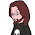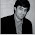## Friday, September 16, 2011

### 007_seasonalrotatinglight_ENGINEERHELP!!!

We don't have an engineer!

If we know our arduino runs at 7-12V, using 200mA, if we want to run off battery power for 8 hours, how many mW do we need it to be rated for?

http://ebtx.com/mech/ampvolt.htm

is it as simple as:

watts= 8 hours (200mA * 12V)

Appreciated!

1.I'm not an engineer.

From my own experience, it depends what the Arduino is doing. Just sitting there is different from blinking an LED, is different from turning a motor. You need to know the Amp Hours you will be using.

http://overlandresource.com/what-is-an-amp-hour-and-how-to-calculate-battery-capacity

http://www.powerstream.com/battery-capacity-calculations.htm

http://easycalculation.com/physics/classical-physics/battery-life.php

2.Thanks John,

Our arduino would only be flipping (electronic) switches so we are assuming minimal power usage. Read that each I/O pin (infrared or proximity sensor x1, voltage sensor, and a few H-switches) usually uses 40-50mA so we arrived at the 200mA measure.

Is it as simple as attaching a unit of time to that, 200mAhours, and then multiplying by run time? In this case arriving at 1600mAh?

3.Indeed, the Amp-hour rating is just the current multiplied by time.
Total energy is that (Amp-hour) multiplied by the voltage at which it is operating.

To give you a sense of scale, the iPhone battery is rated at ~1,500 mA-hrs, or 1.5 Amp-hour.

4.PS: The Li-polymer batteries in most cell phones operate at about 4 or 4.5 volts.

PPS: The typical alkaline, non-rechargeable 9-volt battery that you can attach to your Arduino has a smaller charge capacity – about 0.5 A-hr, but operates at double the voltage. So, altogether it can provide about 2/3 of the energy of a typical cell phone battery.
http://en.wikipedia.org/wiki/Nine-volt_battery